Download Presentation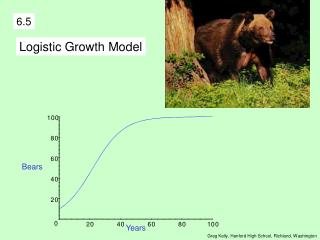6.5

# 6.5 - PowerPoint PPT Presentation

6.5. Logistic Growth Model. Bears. Years. Greg Kelly, Hanford High School, Richland, Washington. We have used the exponential growth equation to represent population growth. The exponential growth equation occurs when the rate of growth is proportional to the amount present.I am the owner, or an agent authorized to act on behalf of the owner, of the copyrighted work described.
Download Presentation## 6.5

An Image/Link below is provided (as is) to download presentation

Download Policy: Content on the Website is provided to you AS IS for your information and personal use and may not be sold / licensed / shared on other websites without getting consent from its author.While downloading, if for some reason you are not able to download a presentation, the publisher may have deleted the file from their server.

- - - - - - - - - - - - - - - - - - - - - - - - - - E N D - - - - - - - - - - - - - - - - - - - - - - - - - -
Presentation Transcript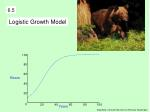6.5

Logistic Growth Model

Bears

Years

Greg Kelly, Hanford High School, Richland, Washington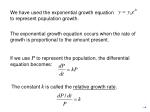We have used the exponential growth equation

to represent population growth.

The exponential growth equation occurs when the rate of growth is proportional to the amount present.

If we use P to represent the population, the differential equation becomes:

The constant k is called the relative growth rate.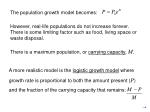The population growth model becomes:

A more realistic model is the logistic growth model where growth rate is proportional to both the amount present (P) and the fraction of the carrying capacity that remains:

However, real-life populations do not increase forever. There is some limiting factor such as food, living space or waste disposal.

There is a maximum population, or carrying capacity, M.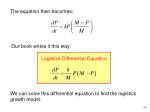Logistics Differential Equation

The equation then becomes:

Our book writes it this way:

We can solve this differential equation to find the logistics growth model.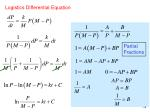Partial

Fractions

Logistics Differential Equation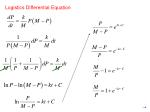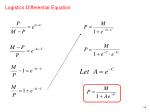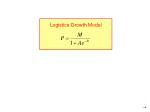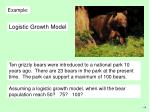Example:

Logistic Growth Model

Ten grizzly bears were introduced to a national park 10 years ago. There are 23 bears in the park at the present time. The park can support a maximum of 100 bears.

Assuming a logistic growth model, when will the bear population reach 50? 75? 100?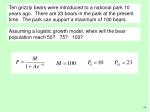Ten grizzly bears were introduced to a national park 10 years ago. There are 23 bears in the park at the present time. The park can support a maximum of 100 bears.

Assuming a logistic growth model, when will the bear population reach 50? 75? 100?Bears

Years

We can graph this equation and use “trace” to find the solutions.

y=50 at 22 years

y=75 at 33 years

y=100 at 75 years

p